Sheri finds the Volume of a Pyramid is 1/3 the Volume of a Cube with the same base and height

Sheri built 3 pyramids out of cm cubes and tried to fit them together to make a cube, but that didn't work.

1. Sheri found 3 pyramids in the Geoblock set that did make a cube. So the volume of the pyramid was 1/3 the volume of the cube with the same base and height.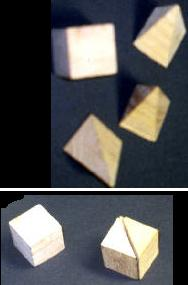2. Then Don asked Sheri to fill a plastic square pyramid with water and see how many it would take to fill the plastic cube. It took 3 pyramids with water to fill the cube. So the volume of the pyramid was 1/3 the volume of the cube with the same base and height. These plastic pieces are from the "Volume Relationship Set"  which was obtained from Ideal School Supply Company.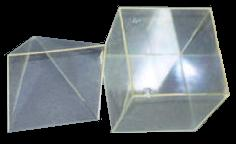3. Don asked Sheri to find the ratio of the volume of a pyramid made with cm cubes to the volume of a cube also made of cm cubes, with the same base and height. The volume of the pyramids were the sum of squares, and the cubes were each a cube number. The picture of the wood pieces is shown below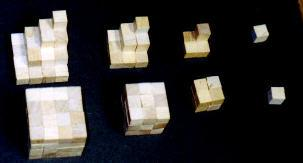Sheri's work to find the sum of the squares, the cubes, and the ratios of the volumes as a fraction and a decimal is shown below. Sheri worked very hard on these!Fine work Sheri!

Using Mathematica, Don and Sheri did the first 4 below; each gives the input, then the second line is the output. Then Don did the rest after Sheri left, but he showed her what he did later.

1. below says: Find the sum of n2, from n=1 to n=3; in other words, add 12 + 22 + 32 = 1 + 4 + 9 = 14

1.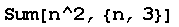14

2. below says: Find the sum of n2, from n=1 to n=100; in other words, add 12 + 22 + 32 + .. +1002  = 1 + 4 + 9 +.. + 10000  = 338350

2.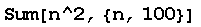338350

3.   100^3

1000000

4.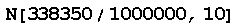0.33835

2. above shows that going to 100 layers of the pyramid the volume is 338350, 3. above shows the volume of the cube of side 100 is 1000000. 4. above shows the ratio of the Volume of the pyramid/ Volume of the cube Sheri found above 0.33835. The command in 4.  tells the computer to divide 338350 by 1000000 and give the answer to 10 digits (it doesn't give all 10 digits if there are zeros on the right). Don made sure Sheri could get the decimal for 1/3 = 0.33333... (and he checked her division for the other ratios).

The ratios Sheri was getting form an infinite sequence

1,   5/8,     14/27,   30/64,   55/125,  91/216, .. , 338350/1000000,...  or as decimals
1, 0.625,  0.518..., 0.468,    0.440,    0.421,..,       0.33835,...

This sequence is a slowly converging sequence; it took 100 terms to reach 0.33835 and is getting closer  to 0.33333... = 1/3. Don used Mathematica to show that the sequence really does go to 1/3.

In 5. below Don used Mathematica to find the sum of the first x squares.

5.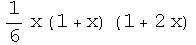In 6. below Don used Mathematica to find the ratio of [the sum of the first x squares/x3 ], which is the ratio of the volume of the pyramid of x layers to the volume of the cube of edge x :

6.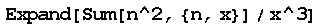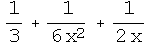In 7. below Don used Mathematica to find the limit of the function above as x goes to Infinity, which is 1/3.

7.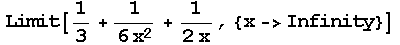Don shows below how he found the rule for the sum of the first x square numbers given in 5 above, without using Mathematica. The numbers in the table below are the numbers Sheri found in her notebook (up to 6 layers). Line 2 in the table means find the sum (y) of the first 2 square numbers (x) = 12 + 22 = 5 (y). Don looked at the differences. (He realized he needed to do guess my rule and their differences with Sheri). Since the 3rd differences are constant, the rule for the sum is a 3rd degree function, and will look like
ax3 + bx2 + cx + d = y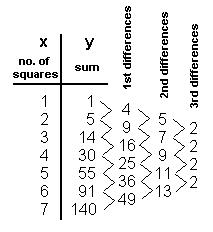In  ax3 + bx2 + cx + d = y , if we put x=1 and y=1,  then x= 2 and y=5,  then x= 3 and y=14, then x= 4 and y=30, we get a system of 4 equations in 4 variables a, b, c, and d. The equation number is in red.
Putting in
x=1,   Eq1 =     a  +      b  +    c  +  d  =   1
x=2,   Eq2 =   8a  +    4b  + 2c  +  d  =   5
x=3,   Eq3 = 27a  +    9b  + 3c  +  d  = 14
x=4,   Eq4 = 64a  +  16b  + 4c  +  d  = 30

To eliminate d:
Eq2-Eq1=Eq5=  7a + 3b + c =  4
Eq3-Eq2=Eq6=19a + 5b + c =  9
Eq4-Eq3=Eq7=37a + 7b + c =16

To eliminate c:
Eq6-Eq5=Eq8=  12a + 2b = 5
Eq
7-Eq6=Eq9=  18a + 2b = 7

To eliminate b
Eq9-Eq8=Eq10=  6a=2, so a = 1/3

To solve for b, put a = 1/3 in Eq8:
12* 1/3 +2b =5
4 + 2b = 5
b = 1/2.

To solve for c, put a = 1/3 and b = 1/2 into Eq5 :
7*1/3 + 3*1/2 + c = 4      Multiply both sides by 6
14   +    9     + 6c = 24
6c = 1
c = 1/6

To solve for d, put  a = 1/3  and   b = 1/2 and  c = 1/6 into  Eq1, and we get
1/3 + 1/2 + 1/6 + d = 1,  and from this
1 + d = 1, so
d = 0

If we now put a = 1/3, b = 1/2, c = 1/6, and d = 0 in the general case
ax3 + bx2 + cx + d = y , we get:
1/3x3 + 1/2x2 + 1/6x  = y    factoring 1/6x from each term, we get
1/6x (2x2 + 3x + 1) and factoring the trinomial, we get

1/6x (2x + 1)(x + 1)   which is the same as 5. above.

Now if we divide 1/3x3 + 1/2x2 + 1/6x by x3 , we get 1/3 + 1/(2x) + 1/(6x2) which is the same as 6. above.
If we take the limit of 1/3 + 1/(2x) + 1/(6x2) as x ->Infinity, the two terms on the right go to zero, so we get 1/3, which agrees with 7. above, and the wooden pieces, and the water filled pyramid and cube, and Sheri's work with the wooden pieces!

Sheri writes 64 using exponents
Sheri solves the quadratic equation x2 - x - 1 = 0
Sheri finds the base for Don's age of   114? = 7110
Sheri uses the quadratic formula to find base for Don's age of   114? = 7110
Sheri finds the measure of an inscribed angle
Sheri uses binary numerals to make the Magic Number Game cards
Sheri changes the shape of a dog using matrices
Sheri reduces the size of a shell using the pantograph
Sheri moves a parabola and finds the equation
Sheri works with the sand pendulum
Sheri figures out a rule for The Tower Puzzle
Sheri starts Trig

To other discoveries

To order Don's materials
Mathman home